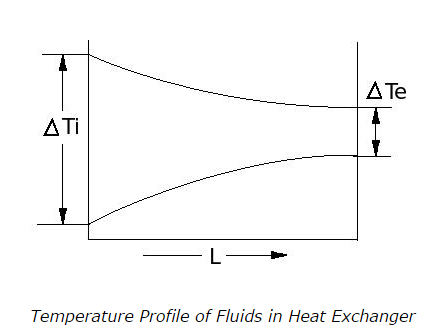# Parallel Flow and Counter Flow Heat Exchangers - MCQs with Answers

## Parallel Flow and Counter Flow Heat Exchangers – MCQs with Answers

1. How is the logarithmic mean temperature difference (LMTD) calculated for heat exchangers?

Where,
ΔTi= temperature difference between hot and cold fluid at inlet of heat exchanger
ΔTe = temperature difference between hot and cold fluid at exit of heat exchanger

a. ln (ΔTi– ΔTe)
b. ln (ΔTe – ΔTi)
c. (ΔTi– ΔTe) / ( ln (ΔTe / Δti))
d. (ΔTi– ΔTe) / ( ln (ΔTi / Δte))

ANSWER: d. (ΔTi– ΔTe) / ( ln (ΔTi/ Δte))

2. Which type of flow in heat exchanger is represented in below diagram?a. Parallel flow heat exchanger
b. Counter flow heat exchanger
c. Cross flow heat exchanger
d. none of the above

ANSWER: a. Parallel flow heat exchanger

3. The arithmetic mean temperature difference for parallel flow heat exchanger is given as

a. ΔTam = (ΔTi– ΔTe)
b. ΔTam = (ΔTi+ ΔTe)
c. ΔTam = (ΔTi– ΔTe) / 2
d. ΔTam = (ΔTi+ ΔTe) / 2

ANSWER: d. ΔTam = (ΔTi+ ΔTe) / 2

4. When is the arithmetic mean temperature difference of heat exchanger used instead of LMTD?

a. when the temperature profiles of two fluids of heat exchanger are sloping downward with curve
b. when the temperature profiles of two fluids of heat exchanger are sloping upward with curve
c. when the temperature profiles of two fluids of heat exchanger are straight
d. none of the above

ANSWER: c. when the temperature profiles of two fluids of heat exchanger are straight

5. How can the arithmetic mean temperature difference and LMTD of a same heat exchanger be compared?

a. the arithmetic mean temperature difference is less than LMTD of a same heat exchanger
b. the arithmetic mean temperature difference is more than LMTD of a same heat exchanger
c. the arithmetic mean temperature difference and LMTD of a same heat exchanger are equal
d. none of the above

ANSWER: b. the arithmetic mean temperature difference is more than LMTD of a same heat exchanger

6. Which of the following temperature difference is safer than other to consider in designing of heat exchangers?

a. Arithmetic Mean Temperature Difference (ΔTam)
b. Logarithmic Mean Temperature Difference (LMTD)
c. Both have nothing to do with safety
d. Other

ANSWER: b. Logarithmic Mean Temperature Difference (LMTD)

7. For the same inlet and exit temperatures of two fluids, the LMTD for counterflow is always

a. smaller than LMTD for parallel flow
b. greater than LMTD for parallel flow
c. same as LMTD for parallel flow
d. unpredictable

ANSWER: b. greater than LMTD for parallel flow

8. For the same heat transfer Q and same overall heat transfer coefficient Uo, surface area required for parallel flow operation is always

a. less than LMTD for counter flow
b. more than LMTD for counter flow
c. same as LMTD for counter flow
d. unpredictable

ANSWER: b. more than LMTD for counter flow

9. In parallel flow heat exchangers,

a. the exit temperature of hot fluid is always equal to the exit temperature of cold fluid
b. the exit temperature of hot fluid is always less than the exit temperature of cold fluid
c. the exit temperature of hot fluid is always more than the exit temperature of cold fluid
d. we cannot predict comparison between exit temperatures of hot fluid and cold fluid

ANSWER: c. the exit temperature of hot fluid is always more than the exit temperature of cold fluid

10. For the same heat transfer Q and same overall heat transfer coefficient Uo, surface area required for cross flow operation is always

a. less than LMTD for parallel flow
b. more than LMTD for parallel flow
c. same as LMTD for parallel flow
d. unpredictable